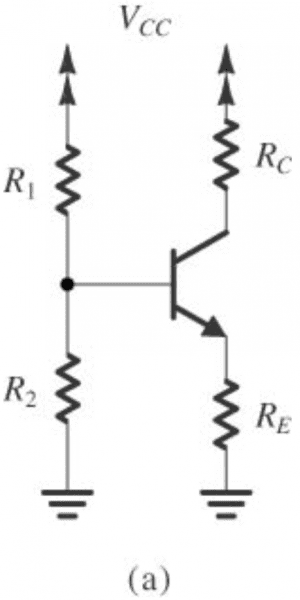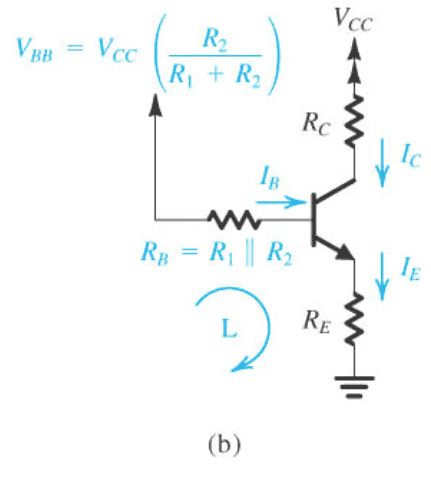# Designing a bias circuit

• Engineering

## Homework Statement

It is required to design the bias circuit as shown in figure for a BJT whose nominal $${\beta}$$ is 100.a.) Find the largest ratio $${(R_B/R_E)}$$ that will guarantee $${I_E}$$ remains within 10% of its nominal value for B as low as 50 and as high as 150.

b.) If the resistance ratio found in a.) is used, find an expression for the voltage $${V_(bb) = V_(cc) *(R_2/(R_1+R_2)) }$$ that will result in a voltage drop of $${V(cc)/3}$$ across $${R_E}$$.

## Homework Equations

$$V(bb) = V(cc) * (R2/ (R1+R2))$$
$$Rb = R1*R2 / (R1+R2)$$

## The Attempt at a Solution

a.) Using the stability factor equation found somewhere else (not sure if it is a relevant equation).

$$S = (1+\beta) (1+ (R_B/R_E))/(1+\beta+(R_B/R_E))$$

For largest ratio, $${\beta}$$ is low and S is high.

$$1.1 = 51 * (1+(R_B/R_E))/(51+(R_B/R_E))$$
$$R_B/R_E = 0.1022$$

b.)
$$V_(bb) - R_B*I_B-0.7-I_E*R_E = 0$$
$$V_(bb) = R_B* (I_E)/(B+1) + 0.7 + V_(cc)/3$$[/B]

#### Attachments

NascentOxygen
Staff Emeritus
b.)
V(bb)−RBIB−0.7−IERE=0​
Hi lisp. http://img96.imageshack.us/img96/5725/red5e5etimes5e5e45e5e25.gif [Broken]

You might be able to use this approach:

Vbb = RB*IE/(β+1) + IE*RE + 0.7

= RE*IE (1 + RB/(RE(β+1))) + 0.7

A decision has to be made: what value to use for β?

Can you post a link to the derivation of the Stability Factor? That might assist someone who may be able to help with that question.

P.S. with the name "lisp", you should be comfortable with lots of nested parentheses!

Last edited by a moderator:
Hi lisp,

I don`t know where your stability formula comes from. Nevertheless, I have used another formula in ealier times, involving simply the max and min values for Ic~Ie:

K=Ic,max/Ic,min=(Numerator N)/(Denominator D) with
N=[(1+B1)/B1 + RB/RE*B1] and D=[(1+B2)/B2 + RB/RE*B2]
with B1=Bmin and B2=Bmax.

Now you can solve for (RB/RE)max. if you know the value of K.
Based on the given 10% Ic variation we have K=(1+0.1)/(1-0.1)=1.222.

Last edited: